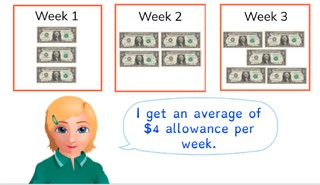Determining the average

# Determining the average

Students learn to determine the average of multiple numbers.

No account needed.8,000 schools use Gynzy92,000 teachers use Gynzy1,600,000 students use Gynzy

## General

The students learn to determine the average.

## Standards

CCSS.Math.Content.6.SP.B.5.C

## Learning objective

Students will be able to determine the average of multiple whole numbers.

## Introduction

Have the students divide the strawberries evenly between the 3 bowls and solve the problem. After that you have the students solve the division problems.

## Instruction

Explain that for determining the average you begin by adding all of the numbers together. Show that you add the numbers 21, 24, 25 and 30 together (21+24+25+30 = 100). Next you divide the total by the number of numbers that you added together to calculate the average (100 ÷ 4 = 25). You can practice solving the following problem together with the students. You can remove the cover over the speech bubble to show the steps as a reminder. For the following problems the students determine the average on their own. Show the table. Explain that you are going to determine the average grade for math tests. First add the numbers from the table together (7 + 6 + 8 = 21). Now divide the total by the number of numbers (21 ÷ 3 = 7). Next you have the students determine the average apples picked per day.

Check whether the students can determine the average, by asking the following question:
- What steps do you take to calculate the average?

## Quiz

The students test their understanding of determining the average through ten exercises. In each of the exercises, they must determine the average of two or more numbers. Some of the exercises are in the form of a story problem, and one exercise has a table.

## Closing

Discuss once again the importance of determining the average. As a closing activity you have the students determine the average daily distance travelled by each snail. Example: The first snail covers the following distances: 4 + 6 + 18 + 4 = 32, 32 ÷ 4 = 8. The snail moves an average distance of 8.

## Teaching tips

Have students that have difficulty with determining the average first practice with the division table of 2. Have them add two numbers together and divide evenly between two students. You can use concrete materials (blocks) to illustrate this.

### The online teaching platform for interactive whiteboards and displays in schools

• Save time building lessons

• Manage the classroom more efficiently

• Increase student engagement